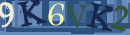Volume 12, Issue 1 And B (Transaction B: Geotechnical Engineering, January 2014)                   IJCE 2014, 12(1 And B): 65-71 | Back to browse issues page

BibTeX | RIS | EndNote | Medlars | ProCite | Reference Manager | RefWorks
Send citation to:Vieira C. A simplified approach to estimate the resultant force for the equilibrium of unstable slopes. IJCE. 2014; 12 (1) :65-71
URL: http://ijce.iust.ac.ir/article-1-748-en.html
Abstract:   (8263 Views)
This paper presents a simplified approach to estimate the resultant force, which should be provided by a retention system, for the equilibrium of unstable slopes. The results were obtained with a developed algorithm, based on limit equilibrium analyses, that assumes a two-part wedge failure mechanism. Design charts to obtain equivalent earth pressure coefficients are presented. Based on the results achieved with the developed computer code, an approximate equation to estimate the equivalent earth pressure coefficients is proposed. Given the slope angle, the backslope, the design friction angle, the height of the slope and the unit weight of the backfill, one can determine the resultant force for slope equilibrium. This simplified approach intends to provide an extension of the Coulomb earth pressure theory to the stability analyses of steep slopes and to broaden the available design charts for steep reinforced slopes with non-horizontal backslopes